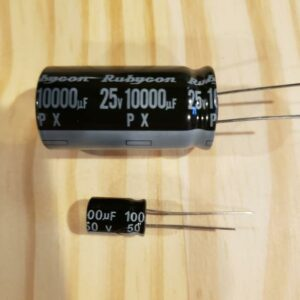Sparkfun Capacitor Kit : \$7.95

• 10pF capacitors (x10)
• 22pF capacitors (x10)
• 100pF capacitors (x10)
• 1nF capacitors (x10)
• 10nF capacitors (x10)
• 100nF capacitors (x25)
• 1µF capacitors (x10)
• 10µF capacitors (x10)
• 100µF capacitors (x10)
• 1000µF capacitors (x10)

Digikey 10000uF (0.01F) capacitor \$3 eachThe energy stored in a capacitor is electrostatic potential energy and is thus related to the charge Q and voltage V between the capacitor plates. A charged capacitor stores energy in the electrical field between its plates.

Capacitance: the ability of a system to store an electric charge. The ratio of the change in an electric charge in a system to the corresponding change in its electric potential.

Capacitor: a device used to store an electric charge, consisting of one or more pairs of conductors separated by an insulator.

Coulomb: the SI unit of electric charge, equal to the quantity of electricity conveyed in one second by a current of one ampere.  The coulomb (symbol: C) is the International System of Units (SI) unit of electric charge. Under the 2019 redefinition of the SI base units, which took effect on 20 May 2019, the coulomb is exactly 1/(1.602176634×10 ) elementary charges. The same number of electrons has the same magnitude but opposite sign of charge, that is, a charge of −1 C.

Farad: the SI unit of electrical capacitance, equal to the capacitance of a capacitor in which one coulomb of charge causes a potential difference of one volt.

1 Farad = 1000mF = 1 million uF = 1 billion nF = 1 trillion pF

m = milli
u = micro
n = nano
p = pico

2 digit markings mean pF: 22 = 22 pF

3 digit marking:  104 = 1 and 0 is 10.  4 represents 10^4 so 10 x 10^4 or 100,000pF = 104:  100,000 pF = 100nF

 Ceramic Marking pF nF uF 10 10 0.01 22 22 0.022 101 100 0.1 102 1000 1 103 10000 10 0.01 104 100000 100 0.1

Let’s look at what a Farad and Ampere-Hour is?

1 F = 1 As/V
1Ah = 3600As

Maintain 1Ah @ 12V you would need?
3600As/12V= 300F

EIA Tolerance Capacitor Marking Code
Letter codeTolerance
Z+80%, -20% – this is used with electrolytic capacitors where the minimum value is the major issue.
M±20%
K±10%
J±5%
G±2%
F±1%
D±0.5%
C±0.25%
B±0.1%
EIA Capacitor Voltage Codes
0G = 4.0VDC1J = 63VDC2D = 200VDC *
0L = 5.5VDC0k = 80VDC2P = 220VDC
0J= 6.3VDC *2A = 100VDC *2E = 250VDC *
1A = 10VDC *2Q = 110VDC2F = 315VDC
1C = 16VDC *2B = 125VDC2V = 350VDC
1E = 25VDC *2C = 160VDC2G + 400VDC *
1H = 50VDC *2Z = 180VDC2W = 240VDC